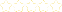• > Precalc-Chapter 2
+# Precalc-Chapter 2

##### Description:
This collection contains all lessons for Chapter 2.

### Your Quiz Points in this Playlist

Total Possible
0
8 Tutorials in this Playlist

#### Precalc Lesson 2.1 "Linear and Quadratic Functions and Modeling"

Rating:(5)

#### Precalc Lesson 2.1 "Linear and Quadratic Functions and Modeling (Vertex Form)"

Rating:(6)

#### Precalc Lesson 2.1 "Linear and Quadratic Functions and Modeling (Standard Form)"

Rating:(0)

#### Precalc Lesson 2.1 "Linear and Quadratic Functions and Modeling (Intercepts Form)"

Rating:(1)

#### Precalc Lesson 2.1 "Linear and Quadratic Functions and Modeling (Writing Functions)"

Rating:(3)

#### Precalc Lesson 2.3 "Polynomial Functions of Higher Degree With Modeling"

Rating:(6)

#### Precalc Lesson 2.4-2.5 "Real and Complex Zeros of Polynomial Functions"